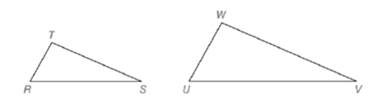Chapter 5.2, Problem 36EElementary Geometry For College St...

7th Edition
Alexander + 2 others
ISBN: 9781337614085

Solutions

Chapter
SectionElementary Geometry For College St...

7th Edition
Alexander + 2 others
ISBN: 9781337614085
Textbook Problem

Given △ R S T , a second triangle ( △ U V W ) is constructed so that U V = 2 ( R S ) , V W = 2 ( S T ) , and W U = 2 ( R T ) . a) What is the constant value of the ratios U V R S , V W S T , W U R T ? b) Using intuition (appearance), does it seem that △ U V W is similar to △ R S T ?To determine

a)

The constant value of the ratios UVRS,VWST,and WURT.

Explanation

Definition:

Similarity between Two polygons:

Two polygons are similar if and only if when all pairs of corresponding angles are congruent and all pairs of corresponding sides are proportional

Given:

It is given that UV=2(RS),VW=2(ST),and WU=2(RT).

Calculation:

Divide by RS in both side of the equation UV=2(RS), we get

UVRS=2(RS)RS=2

Divide by ST in both side of the equation VW=2(ST), we get

To determine

b)

Whether it seems that ΔUVW is similar to ΔRST.

Still sussing out bartleby?

Check out a sample textbook solution.

See a sample solution

The Solution to Your Study Problems

Bartleby provides explanations to thousands of textbook problems written by our experts, many with advanced degrees!

Get Started

Find more solutions based on key concepts